# Deriving and verifying the uncertainty on conversion rate predictions

Posted by on August 12, 2019

For the past few weeks I’ve been working on building a machine learning model that can estimate the probability that a customer will convert from the free trial to a paid subscriber. In practice, I combine the predictions from this model for cohorts of companies, which are defined by their acquisition channel and acquisition month, and so a method is required for calculating the conversion rate uncertainties for each cohort.

## Uncertainty matters

The uncertainty on an observed value quantifies how confident we are that the observation wasn’t a fluke1. In this context, providing the value of a conversion rate without also providing the uncertainty on that value is dangerous and open to misinterpretation. For example, an acquisition channel with a predicted conversion rate of 30%, with an uncertainty of 2% may be more successful than one with a predicted conversion rate of 90% but with an uncertainty of 80%! If uncertainty values were missing we would be misled by the prediction values and come to the conclusion that the second acquisition channel is much better than the first.

The sample of probabilities of subscription is an example of the occurrence of the little-known Poisson-Binomial distribution2 in a real-life process. In this post I will cover how I derived the uncertainty value for the conversion rate analytically, using the Poisson-Binomial distribution, and how I verified its accuracy numerically using a Monte Carlo simulation3.

## Bernoulli Distribution

For each company the model outputs a probability of subscriptionwhich corresponds to a random variable. This is an example of a Bernoulli random variable which has only two possible outcomes – success (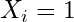) or failure (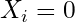). In this case, success is subscription which has probability.

The expected value of the random variableis:and the variance: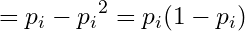## Poisson-Binomial Distribution

For a cohort of companies, we have a sequence of not necessarily identically distributed Bernoulli random variables, each with a probability of success. To predict the conversion rate of a cohort we need to estimate the number of companies that are expected to subscribe. Let this be denoted by, which is the sum of all the Bernoulli random variableswhereis the number of companies in the cohort.

Therefore, the expected number of companies to subscribe in the cohort is: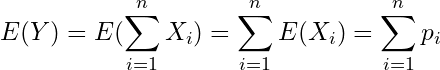Since we assume each company subscribes independently of each other, the Bernoulli random variables are independent and we can simply sum their variances to calculate the variance of: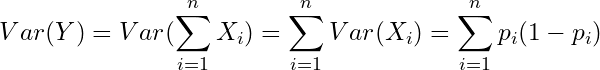The sum of independent non-identical Bernoulli trials follows the Poisson-Binomial Distribution. The more well-known Binomial Distribution is a special case of the Poisson-Binomial Distribution where all Bernoulli random variables have equal probabilities of success.

Letdenote the conversion rate. For a cohort ofcompanies, we have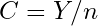. Therefore: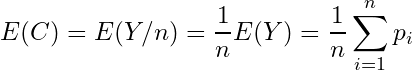This is just the arithmetic mean of the probabilities.

The uncertainty on the conversion rateis its standard deviation, which is the square root of the variance.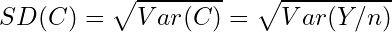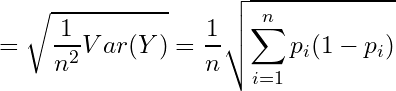## Calculating the conversion rate and uncertainty using python

For this we are using numpy

import numpy as np

For the purposes of this example we are going to create an one-dimensional array of 1,000 fake randomly generated probabilities of subscription.

np.random.seed(0)
n = 1000
probs = np.random.rand(n)

We can calculate the expected conversion rate along with the relevant uncertainty (standard deviation) based on the formulae derived above:

conversion_rate = np.mean(probs)
uncertainty = np.sqrt(np.sum(probs*(1-probs))) / n

For this example the conversion rate is found to be 0.49592 and the uncertainty 0.01287

## Verifying the results using a Monte Carlo simulation

The general idea of a Monte Carlo simulation is to repeat random sampling multiple times to (in this case) estimate the conversion rate and its uncertainty. For each company I took a random number and if the probability of subscription of the company is greater than that number, I consider the company subscribed, otherwise not subscribed. After this process is completed for all companies I calculate the conversion rate and store it in a list. I repeat this process 10,000 times and then I calculate the mean of all the conversion rates as the estimated conversion rate. The uncertainty is the standard deviation of this set of conversion rates.

MC_conversion_rates = []
for i in range(10000):
thresholds = np.random.rand(n)
subscribed = probs > thresholds
MC_conversion_rates.append(sum(subscribed) / n)
MC_conversion_rate = np.mean(MC_conversion_rates)
MC_uncertainty = np.std(MC_conversion_rates)

The estimated conversion rate from the Monte Carlo simulation is 0.49592 and the uncertainty of this is 0.01289, which is consistent with the result derived above.

The next steps for my project are to integrate the subscription probability predictions into a cloud-based machine learning pipeline. This pipeline will generate new predictions each day, allowing us to surface the predictions and uncertainties to stakeholders in the business, to help optimise our marketing efforts in different acquisition channels.

## References

1. Holdgraf, C. (2014). The importance of Uncertainty. [Blog] Berkeley Science Review. Available from: https://berkeleysciencereview.com/importance-uncertainty/ [Accessed 8th August 2019]
2. Wang, W. H. (1993). On the number of successes in independent trials. Statistica Sinica. 3: 295-312. Available from: http://www3.stat.sinica.edu.tw/statistica/oldpdf/A3n23.pdf [Accessed 8th August 2019]
3. Pease, C. (2018). An overview of Monte Carlo methods. [Blog] Towards Data Science. Available from: https://towardsdatascience.com/an-overview-of-monte-carlo-methods-675384eb1694 [Accessed 8th August 2019]